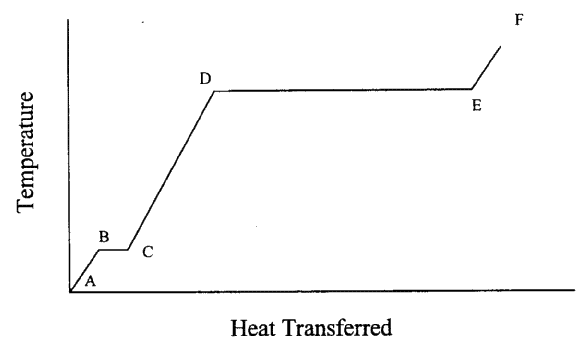## University of California, San Diego Physics 1b - Thermodynamics, Electricity & Magnetism

 H. E. Smith Spring 2000Physics 1B - Tutorial #21. Very cold ice is placed in a closed container. Heat is then applied to the container. Below is a graph of the temperature inside the container plotted against the amount of heat delivered to the system.• Describe what is happening to the substance inside the container from point A to point F.
• Put temperature scales in Celsius on the vertical axis.
• If the heating rate is constant (dQ/dt = constant), compare the time it takes to heat from B to C to the time it takes to heat from D to E.

2. Materials A and B have equal densities. But A has twice the specific heat of B. You have two 100 g cubes made of materials A and B.
• Cube A, initially at 0°C, is placed in good thermal contact with cube B, initially at 100°C. Both are placed in a well-insulated container. What is the final temperature?
• Cubes A and B are both heated to 200°C, and then placed in room temperature environment. Which one cools faster?

3. A thermometer is laid out in direct sunlight. Does it measure the temperature of the air, of the sun, or of something else?

4. The inside of an oven is at 400° F. But you can still put your hand in the oven as long as you don't touch anything. But since the air inside the oven is also at 400°F, why isn't your hand burned just the same? What if you leave your hand in the oven for a long time (e.g. 2 hours)?

5. While jogging, an average 65 kg student generates thermal energy at a rate of 300 W (about 0.4 horse power). To maintain a constant body temperature of 37°C, this energy has to be dissipated by perspiration or other mechanisms.
• If these mechanisms failed and the heat couldn't flow out of the student's body, for how long could a student run before irreversible body damage could occur? (Protein structures in the body are irreversibly damaged at 44°C or above. The specific heat capacity of a typical human body is 3480 J/kg.K, slightly less than that of water.)
• If the heat loss is by sweat evaporation only, calculate the volume of water evaporated per minute (latent heat Lv = 539 cal/g). How many liters of water are needed to compensate the water loss of a two hour jog? What other heat loss mechanisms do you think may be important for a runner?Physics 1B HomeTutorials

Gene Smith